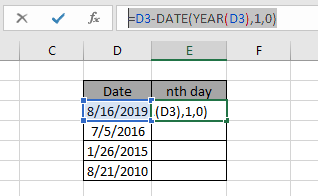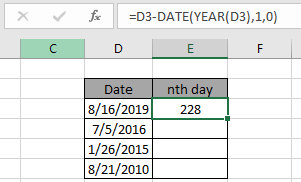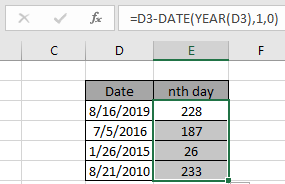# Get Nth Day From Date of Year in Excel

In this article, we will learn about how to get to the nth day of year in Excel. It is like getting serial number of a date in its year.

Scenario:
Let's say we have a date. Now we need to find out what day of the year it is. To get the date of year in excel we will make a formula using the DATE & YEAR function. We will use the serial number attribute of date value in Excel. We will get the last date of the previous year and subtract the result form the given date value.

Generic Formula:

= date - DATE (YEAR ( date ), 1 , 0 )

date : given date value

All the above explained procedures might be confusing. So clear all the doubts via the example shown below.

Here we have some random data values and we need to find the nth day of year from the date value.Use the formula:

= D3 - DATE ( YEAR ( D3 ) , 1 , 0 )

Explanation:

1. YEAR function returns the year value of the given date.
2. DATE function takes the argument and returns the last date of the previous year.
3. The subtraction operator takes the difference between the two and returns the elapsed date.Use the above function formulas to get the days between dates in Excel.As you can see, the formula works fine as it returns 228 as a result. Now use drag drop down till the dataset dates.Here are the days from the date of the year calculated using the formula.

We can calculate the same result using one more logic. If we subtract the given date value from the first date of the same year and add 1 ( +1 ).

Generic formula:

= date - DATE ( YEAR ( date ) , 1 , 1 ) + 1

You can use any of the formulas as both return the same  result.

Note: DO NOT give the data argument directly to the formula as the Excel formula doesn't necessarily understand the date value and returns error. Provide the date argument using the DATE function or using the cell reference option.

Hope you understood how to Get nth day from date of year in Excel. Explore more articles on Excel Date & TIME functions here. Please feel free to state your query or feedback for the above article.

Related Articles

Calculate age from date of birth

Calculate days, years and months from certain date

How to use the EDATE function in Excel

SUM if date is between

Vlookup by Date in Excel

Popular Articles

50 Excel Shortcut to Increase Your Productivity

Edit a dropdown list

Absolute reference in Excel

If with conditional formatting

If with wildcards

Vlookup by date

Join first and last name in excel

Count cells which match either A or B

Terms and Conditions of use

The applications/code on this site are distributed as is and without warranties or liability. In no event shall the owner of the copyrights, or the authors of the applications/code be liable for any loss of profit, any problems or any damage resulting from the use or evaluation of the applications/code.